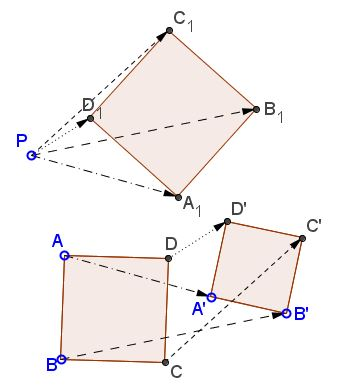# Two Squares and Another SquareWhat is that about? A Mathematical Droodle

Explanation### Explanation

The applet suggests the following statment:

Given two squares ABCD and A'B'C'D' and a point P, let A1 = P + AA', B1 = P + BB', C1 = P + CC', and D1 = P + DD'. Then A1B1C1D1 is a square.This is problem 46a from Yaglom. One solution follows from a more general statement about two directly similar figures.

### References

1. I. M. Yaglom, Geometric Transformations II, MAA, 1968